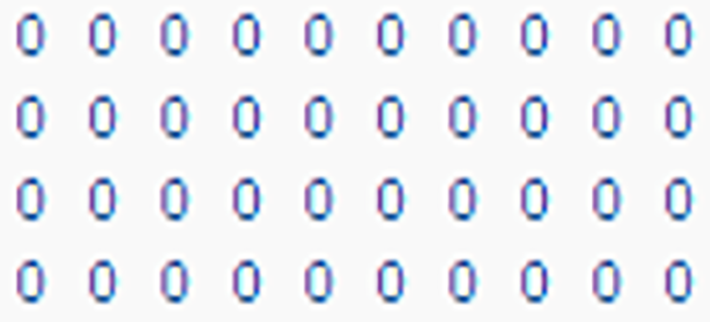# Leading and trailing zeros in ABAP

000 Everything you need to know about handling zeros in ABAP 00Leading and trailing zeros are any zero digits occurring before the first and after the last nonzero digit in a number sequence, respectively.

In SAP, most alphanumerical fields are stored in the database with leading zeros, fully taking up the defined length of the field. When the field is shown to the end-user, however, the leading zeros are left out.

SAP handles this data normalization process by using so-called conversion exits to convert a value from an external (output) format to an internal (input) format.

Adding or removing leading zeros is a common requirement in the daily life of an ABAP developer. As the syntax has evolved over the years, more and more possibilities became available to realize said requirement.

## Conversion exit examples

The examples below use the order number data element `AUFNR`, which has data type CHAR and length 12.

``````DATA(lv_order_number) = CONV aufnr( 12345 ).
``````

Opening the domain of the field we want to convert in the ABAP Dictionary shows us we can use the ‘ALPHA’ conversion routines. Developers can use these function modules to add and remove leading zeros to most numeric/ID-type fields.

### Function modules

In days gone by, the function modules for the ALPHA conversion routine were the best way to add and remove zeros.

``````DATA(lv_order_number) = CONV aufnr( 12345 ).
WRITE |{ lv_order_number }|.    "Output: 12345

CALL FUNCTION 'CONVERSION_EXIT_ALPHA_INPUT'
EXPORTING
input  = lv_order_number
IMPORTING
output = lv_order_number.

WRITE |{ lv_order_number }|.    "Output: 000000012345

CALL FUNCTION 'CONVERSION_EXIT_ALPHA_OUTPUT'
EXPORTING
input  = lv_order_number
IMPORTING
output = lv_order_number.

WRITE |{ lv_order_number }|.    "Output: 12345
``````

### Short form using string templates

Significant improvements to string processing in recent ABAP releases have made zero handling code less verbose. For example, since the arrival of string templates in ABAP 7.4 and the embedded ALPHA expression in ABAP 7.2 it has been possible to achieve the result of the ALPHA conversion in a single line.

``````DATA(lv_order_number) = CONV aufnr( 12345 ).
WRITE |{ lv_order_number }|.    "Output: 12345

lv_order_number =  |{ lv_order_number ALPHA = IN }|.
WRITE |{ lv_order_number }|.    "Output: 000000012345

lv_order_number =  |{ lv_order_number ALPHA = OUT }|.
WRITE |{ lv_order_number }|.    "Output: 12345
``````

### Inline declaration with constructor operator CONV

One can even declare a variable and pad it with leading zeros in a single line, eliminating the need for possible helper variables.

``````DATA(lv_order_number) = CONV aufnr( |{ '12345' ALPHA = IN }| ).
WRITE |{ lv_order_number }|.    "Output: 000000012345
``````

## Other examples

### Removing leading zeros with SHIFT

The SHIFT statement can be used to remove zeros strings.

``````DATA(lv_order_number) = CONV aufnr( |{ '12345' ALPHA = IN }| ).
WRITE |{ lv_order_number }|.    "Output: 000000012345

SHIFT lv_order_number LEFT DELETING LEADING '0'.
WRITE |{ lv_order_number }|.    "Output: 12345
``````

### Add trailing zeros with OVERLAY

The OVERLAY statement can be used to pad a string with trailing zeros.

``````DATA(lv_order_number) = CONV aufnr( 12345 ).
WRITE |{ lv_order_number }|.    "Output: 12345

"Helper variable of type string, without any leading or trailing zeros
DATA(lv_order_number_string) =  |{ lv_order_number ALPHA = OUT }|.
OVERLAY lv_order_number_string WITH '000000000000'.
WRITE |{ lv_order_number_string }|.    "Output: 123450000000
``````